Programming Example: Fraction Math

This topic presents a programming example designed to incorporate the concepts examined up to this point. The vehicle for this example is a "fraction math" application, which allows the user (such as a grade school child, or parent of that child trying to help their kid with the homework) perform one of the four basic arithmetic operations (addition, subtraction, multiplication, or division) on a pair of fractional numbers.

The program will allow the user to choose one of the four operations (add, subtract, multiply, or divide).  Text boxes will be presented for the user to enter in the two terms of the problem (the problem will be limited to two "terms", or items to be operated on).  Each term can be either a fraction (for example,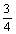), a mixed number (for example, 2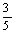), or a whole number (for example, 4).  Therefore, each term will consist of three textboxes: one for the whole number portion of the problem (if any), one for the fraction numerator, and one for the fraction denominator.

After the user has entered the data for the problem and clicks the "Calculate" button, the program will display the result of the calculation. The result is expressed as a fraction, reduced to lowest terms.

The form also contains a "Clear" button, which allows a user to perform a new calculation, and an "Exit" button, which allows a user to exit the program.  The form uses appropriate tabbing order and access keys.

In case you are a little rusty, here is a brief review of fraction math:

First, if there is a mixed number in the problem, convert this to a fraction by multiplying the integer portion of the number by the denominator of the fraction, then add the numerator to this result.  This result becomes the new numerator, and the integer portion is discarded.

For example, 2becomes.

#### Addition

After converting any mixed numbers to fractions, you must find a common denominator for both fractions.  Adjust the numerators appropriately for the new denominators by multiplying the numerator by the same number you used to get the new denominator, add the numerators together and place that result over the common denominator, then convert the result back to a mixed number.

Sample problem:

2+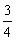=          ?

2+=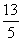+=+==          3#### Subtraction

(Similar to addition.) After converting any mixed numbers to fractions, you must find a common denominator for both fractions.  Adjust the numerators appropriately for the new denominators by multiplying the numerator by the same number you used to get the new denominator, subtract the numerators and place the difference over the common denominator, then convert the result back to a mixed number.

Sample problem:

2-    1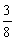=           ?

2- 1=-=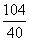-=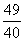=          1#### Multiplication

Convert any mixed numbers to fractions.  Multiply the two numerators; that product becomes the new numerator.  Multiply the two denominators; that product becomes the new denominator.  Convert the final result back to a mixed number.

Sample problem:#### Division

(Similar to multiplication.)  Convert any mixed numbers to fractions.  Switch the numerator and the denominator of the second factor.  Proceed as in multiplication.

Sample problem:The programming solution consists of two forms (a splash screen and the main form).

When the user first runs Fraction Math, the following "splash screen" briefly appears:The main screen then appears, where the user can select which operation they wish to perform ("Add" is the default) and key in the numbers that make up the problem:When the user clicks the Calculate button, the solution is displayed. Note that some of the intermediate results of the solution were displayed.  This was a design choice; others might have opted to just display the final result.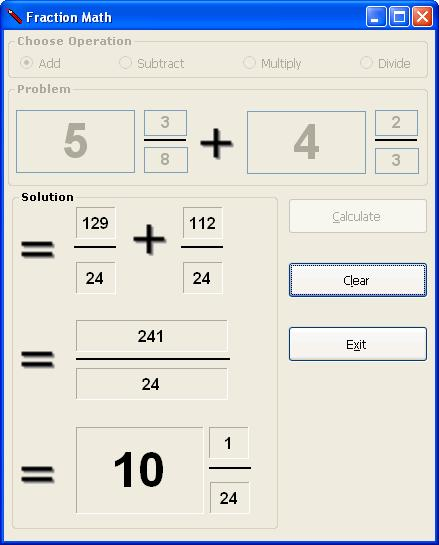This program incorporates the following:

• Radio buttons
• Use of a splash screen
• Use of images (for the splash screen, for the mathematical symbols (add, subtract, multiply, divide, equals), and for the main form icon)
• Simulation of control arrays for the textboxes and labels

Note: For the lines that appear between the numerator and denominator of the fractions, labels were used. You can use a label to create a horizontal line by setting these properties as follows:

Autosize = False

BorderStyle = FixedSingle

Text = (none)

Size: Height = 2

Download the VB project code for the example above here.